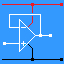RANDOM PAGE

SITE SEARCH

LOG
IN

HELP

This is the AQA version closing after June 2019. Visit the the version for Eduqas instead.

Here is another way of using or thinking about the AND gate.

Input A carries data. Input B is used to open or close the gate.

• If B = 1, the gate is open and the data passes through.
• If B = 0, the gate is closed and the output is zero.

Here is an example of bit masking using the idea above.This diagram shows a hardware bit mask.

The top four gates are open and the input data is copied to the output.

The bottom four gates are closed and the output is always zero.

Circuits like this are used to select wanted bits and to ignore unimportant bits.

Software bit masks are possible too. For example the program below reads data from PORTA. If pins D4, D5, D6 and D7 are not being used, they need to be ignored. The code below achieves this.

This software is equivalent to the hardware in the diagram.

```    MOVRW   PORTA   ' Input from PORTA
ANDW    0x0F    ' Ignore bits 4, 5, 6, and 7
```

The Internet uses 32 bit IP addresses (IPv4). Internet routers are devices that decide how to route Internet traffic. The left hand bits in an IP address are used to identify the network. Routers use these "network bits" to make routing decisions. The right hand bits are used to identify the host (a computer, server, printer, etc). Routers are not interested in the "host bits".

The number of network and host bits varies from network to network. A method is needed to tell the routers how many bits are used for the network part of the IP address. The subnet mask is used for this. This is a nice example of a bit mask. The subnet mask is ANDed with the IP address. 32 AND gates are needed. The result is the network address that the router needs for its routing decisions. Where the subnet mask contains ones, the IP address bits are passed through the AND gates. Where the subnet mask bits are zero, the IP address bits are blocked by the AND gates. This hardware method is much faster than the same process done using software.

 IP Address 192.168.33.17 11000000 10101000 00100001 00010001 Subnet Mask 255.255.255.0 11111111 11111111 11111111 00000000 IP Address AND Subnet Mask 192.168.33.0 11000000 10101000 00100001 00000000

Or gates can be used for bit masking too.

Input A carries data and input B is used to open or close the gate.

• If B = 0, the gate is open and the data is passed through.
• If B = 1, the gate is closed and the output is one.

Input A carries data and input B is used to mask the data.

• If B = 0, the input data is copied to the output.
• If B = 1, the input data is inverted (NOT Rule) and passed to the output.

reviseOmatic V3     Contacts, ©, Cookies, Data Protection and Disclaimers Hosted at linode.com, London